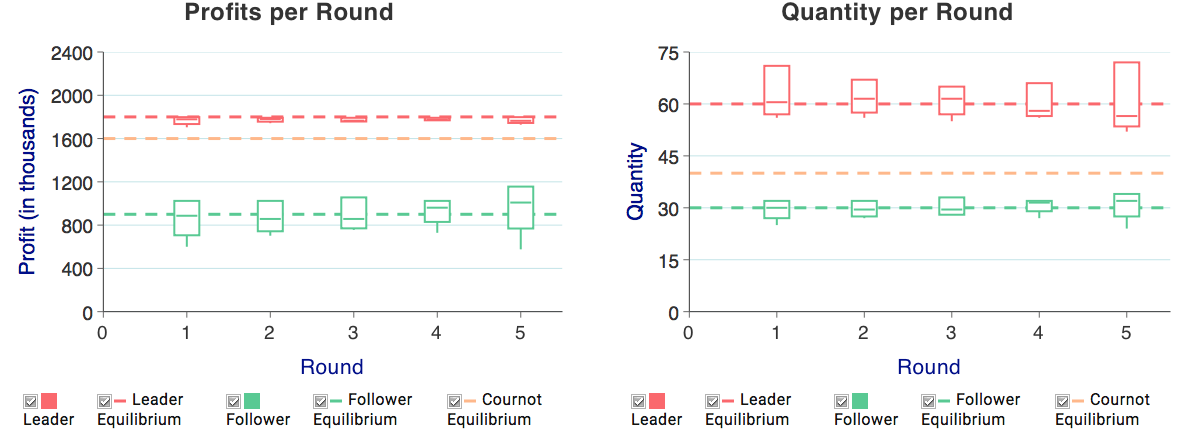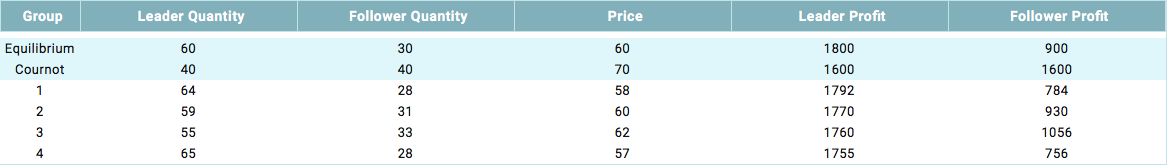MobLab
Guides
Aa

# Stackelberg Competition

## Game Description

In each round, each of two firms sequentially chooses output of a homogenous good (direct flights). The firm choosing first (LeadAir) knows that the second-moving firm (FollowAir) will learn the leader’s output choice before choosing its output. Market price is determined by a linear demand curve. At the end of each round, each firm learns market production, market price, and both firms’ profits.

### Learning Objective 1: Subgame-perfect Nash Equilibrium

We can find the subgame-perfect Nash equilibrium with backwards induction. In the Stackelberg model, we assume that each leader output induces a follower best-response output, and the leader chooses the most profitable leader output under this assumption.

### Learning Objective 2: First-mover Advantage in Quantity Competition

If the first-mover can credibly commit to increased output (e.g., with an irrevocable choice), its equilibrium profits increase relative to the simultaneous-move (Cournot) equilibrium. The follower both produces and earns less relative to the Cournot equilibrium.

## Brief Instructions

With repetition, you should expect qualitative convergence to the subgame-perfect Nash equilibrium as first-movers learn to increase production (relative to Cournot) and it is easy for second-movers to best respond. Repetition is likely going to be more effective without fixed pairings. To do so, set Number of Rounds to 1. When all groups are done, click Finish, then Replay. Choosing Random randomly assigns both role and pairings, while Fixed Role randomly assigns pairings only. For further details on replay options, click here.

Market demand is P(Q)=150-qL-qF, and each firm's constant marginal cost of production is 30. We chose these parameters to induce easy-to-solve integer equilibria for both Stackelberg (qL=60; qF=30) and Cournot (qi=40).

## ResultsFigure 1: Outcomes Across Rounds For a multi-round (Number of Rounds >1) game, the graphs available under Go To: Multi Round Summary enable you to quickly show convergence to the equilibrium prediction. The graph on the left displays, for each round, box-and-whiskers plots of leader and follower profits. The graph on the right displays the same for quantities. (The boxes span the 25th and 75th quartiles, with a line indicating the median. The whiskers extend to the the extreme values.)Figure 2: Outcome Per-group Table

For each round, we also provide a table and a graph. The table (Figure 2) allows you to compare each group’s outcome (each firm’s quantity and profits and market price) to two benchmarks: the equilibria with sequential choice (Stackelberg) and with simultaneous choice (Cournot).

In each round, we also plot each group’s quantity choices in a graph which depicts both the leader’s and follower’s best-response functions. This may help teach the logic of the Stackelberg equilibrium. Most follower’s do in fact best respond, meaning that most group’s choices will fall on the follower’s best-response function. You can make the point that the leader is effectively choosing a quantity combination along the follower’s best-response function, and therefore wants to choose the leader quantity resulting in the highest profit for the leader.

Note that you can dynamically choose which elements to include in the graph. For example, to remove the leader’s best response function, uncheck the appropriate box in the legend.

Finally, if you replayed a particular game (e.g., a series of one-round games), display the results of the last game. You can then look at previous games in the series by clicking the button next to View:.

## Robot Play

Our robot (i.e., an automated player) strategies for each role are the following:

• Leader: Assuming demand curve $P=A-B(q_1+q_2)$, with constant marginal cost $C$, the equilibrium quantity for the leader is $q_1=\frac{A-C}{2B}$. This strategy will pick a quantity from a binomial distribution centered around the equilibrium quantity.
• Follower: A robot follower strategy always chooses the best response. Given demand function $P=A-B(q_1+q_2)$ with constant marginal cost $C$, the response is given by $q_2=\frac{A - C}{2B-\frac{q_1}{2}}$.